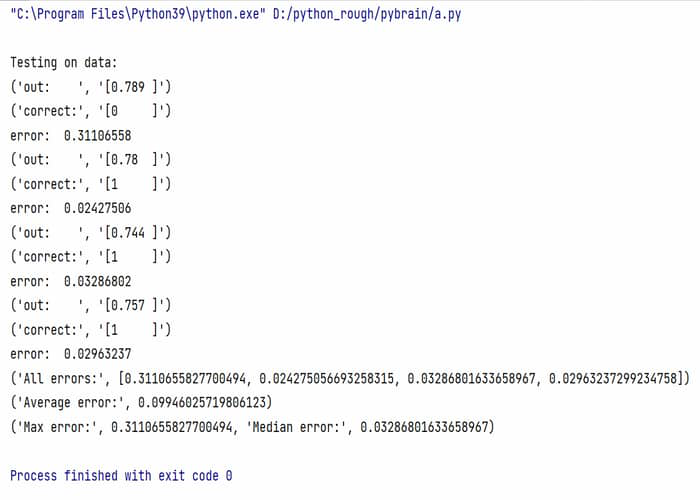# What is Supervised Dataset in PyBrain?

• Difficulty Level : Expert
• Last Updated : 21 Feb, 2022

In this article, we will be looking at the various uses and functionality of the supervised dataset in Pybrain.

A Dataset is a collection of data where we do give the list of values to each member belonging to the dataset. A supervised dataset following supervised learning has input and output fields. In this example, we will learn how to use a Supervised Dataset with PyBrain.To install Pybrain refer to How to Install PyBrain?

Let’s create a OR table where we have an input in form of a 2-D array and we get one output.

```0 or 0 -> 0
0 or 1 -> 1
1 or 0 -> 1
1 or 1 -> 1```

Libraries used:

• buildNetwork is a simple way to create networks that are composed of Modules connected with Connections.
• TanhLayer: After building a network we have to use some layer either TanhLayer or SoftmaxLayer. We will be using TanhLayer in our example.
• SupervisedDataSet: We have to set two values to input and target fields.
• BackpropTrainer: For training according to the supervised dataset

Example:

In this example, after building a network we will create two datasets one for training and another for testing.

## Python

 `# importing buildNetwork  ``# from pybrain.tools.shortcuts``from` `pybrain.tools.shortcuts ``import` `buildNetwork`` ` `# importing TanhLayer``# from pybrain.structure``from` `pybrain.structure ``import` `TanhLayer`` ` `# importing SupervisedDataSet``# from pybrain.datasets``from` `pybrain.datasets ``import` `SupervisedDataSet`` ` `# importing BackpropTrainer``# from pybrain.trainers``from` `pybrain.supervised.trainers ``import` `BackpropTrainer`` ` `# creating a network with TanhLayer``# two input, two hidden and on output``network ``=` `buildNetwork(``2``, ``2``, ``1``, bias``=``True``, hiddenclass``=``TanhLayer)`` ` `# Creating a dataset for training``# 2 output``# 1 input``or_train ``=` `SupervisedDataSet(``2``, ``1``)`` ` `# Creating a dataset for testing.``or_test ``=` `SupervisedDataSet(``2``, ``1``)`` ` `# Adding sample input``# 0 or 0 -> 0``# 0 or 1 -> 1``# 1 or 0 -> 1``# 1 or 1 -> 1``or_train.addSample((``0``, ``0``), (``0``,))``or_train.addSample((``0``, ``1``), (``1``,))``or_train.addSample((``1``, ``0``), (``1``,))``or_train.addSample((``1``, ``1``), (``1``,))`` ` `# Similarly adding samples for or_test``or_test.addSample((``0``, ``0``), (``0``,))``or_test.addSample((``0``, ``1``), (``1``,))``or_test.addSample((``1``, ``0``), (``1``,))``or_test.addSample((``1``, ``1``), (``1``,))`` ` `# Training network with dataset or_train.``trainer ``=` `BackpropTrainer(network, or_train)`` ` `# 1000 iteration on training data.``for` `iteration ``in` `range``(``1000``):``  ``trainer.train()``   ` `# Testing data``trainer.testOnData(dataset``=``or_test, verbose ``=` `True``)`

Output:My Personal Notes arrow_drop_up